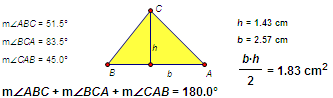﻿ Objects > Calculations

# CalculationsCalculations are mathematical expressions that relate one or more terms — such as measurement — by arithmetic. For example, after you measure the interior angles of a triangle, use Sketchpad’s Calculator to find the sum. Similarly, measure the base and height, and use the Calculator to find the area. You can define a calculation using measurements in your sketch as well as various mathematical operators, built-in functions, and even functions that you’ve defined yourself.

As you change the measurements on which a calculation depends, the calculated result changes accordingly.

Like all value objects, calculations can be used to dynamically control other objects in your sketch.

By observing calculations you can gain valuable insights into mathematical relationships.

To create a calculation, choose

To edit an existing calculation, double-click it with the Arrow tool or select it and choose

To change a calculation into a parameter, edit the calculation to make it a simple numeric value with no operators or functions. (It can be dimensionless, or it can have angle or distance units.)

To change a calculation’s precision and how it’s displayed, select the parameter and choose